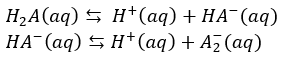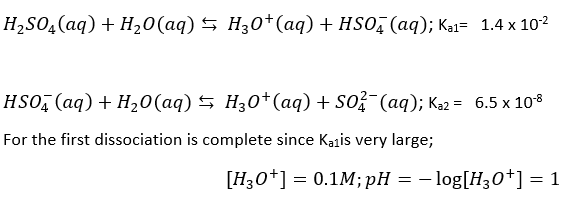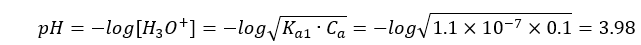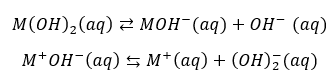# Ionization of a polybasic acid

Equilibrium: Ionization of a polybasic acid

A polybasic acid or polyprotic acid is a type of acid that has more than one replaceable hydrogen atoms. This means that the acid can form more than one ionizable ion per molecule. Recall that an acid is a proton donor.

The general formula for a dibasic acid:

H2A = fully acidic form

HA = intermediate form; amphoteric

A2- = fully basic or fully deprotonated form

The ionization reaction for a dibasic acid is as follows:From these reactions, we can observe that it takes two steps to fully remove the H+ ion from a dibasic acid. This reaction produces two equivalence points or stoichiometric points. Note that, the equivalence pointis the point during an acid-base titration in which equal amounts of acid and base reacted. From the titration (pH-Volume) graph we can notice exactly two equivalence points. The primary reaction involves the dissociation of H2A and H3O+ in the solution comes mainly from the first dissociation step.Figure 1: Titration curve for a strong base.

Polybasic acids have several equilibrium constants depending on the number of protons they can donate. For example, phosphoric acid contains three ionizable hydrogen atoms which mean it has 3 ionization constants.

H3PO4 + H2O H3O+ + H2PO4 ; Ka1= 7.2 x 10-3

H2PO4+ H2O H3O+ + HPO42 ; Ka2 = 6.3 x 10-8

HPO42+ H2O H3O+ + PO43 ; Ka3 = 4.2 x 10-13

Ka1> Ka2> Ka3

The overall dissociation constant can be presented as:

K = Ka1x Ka2x Ka3

Generally, it is observed that higher order ionization constants are smaller than lower order ionization constants for a polybasic acid. The reason being that it is more difficult to remove one +ve charge hydrogen ion from the -ve ion due to higher electrostatic force. Likewise, removing a proton from H2PO4 is more difficult than removing one from H3PO4.

There are two types of polybasic acids:

1. With the first dissociation being complete (Ka1 very large)
2. Withthe first dissociation being partial (K relatively small a1)

Consider 0.1 M of sulphuric acid to discuss case 1This shows that sulphuric acid is a strong acid

Now consider 0.1 M of hydrogen sulphide to discuss case 2

For H2S, the first dissociation is in a similar format as case 1This shows that hydrogen sulphide is a weak acid. Likewise, large Ka1 corresponds to strong acid and large Ka2 corresponds to weak acid.Figure : Examples of polybasic acids at 298 K

Alternatively, polyacidic bases also exist that can accept multiple hydrogen atoms and dissociate into its constituent ions. The number of hydroxide ions formed is corresponding to the capacity of acceptance of protons.Recall that a base is a proton acceptor.

For example: